# SEGMENT TREES

1. update i value: Update element of array A at index i with value.
2. query l r: Print the sum of the array A from index l to index r.
1. update i value: Update the element in the array A at index i with value.
2. query l r: Print the min of the array A from index l to index r.
1. UPDATE i value: Update the element in the array A at index i with value.
2. QUERY l r: Print the gcd of the array A from index l to index r.

# When should I use a segment tree?

1. The function f must be associative:
i.e. f(a,b,c) = f(a,f(b,c)) = f( f(a,b),c). The functions in the above problems are addition, min, and gcd which are associative.
For addition let f(a,b) denote a+b.
f(a,b,c) = a+b+c = a+(b+c) = f(a,f(b,c)) = (a+b)+c = f(f(a,b),c).
We can prove similar results for min(a,b) and gcd(a,b) as well.
We can extend this result to a range [l,r] as follows:
Let g(l,r) denote the application of the binary function f(a,b) on all the elements array A in the range l to r.
thus, g(l,r) = f(A[l],A[l+1],A[l+2]…..A[r])
also as f(A[l],A[l+1],A[l+2]…..A[r]) = f(A[l..k],f(A[k+1..r]) for some k in the range [l,r].
this makes g(l,r) = g(g(l,k),g(k+1,r)).
2. The answer of an interval [l,r] can be derived from the answer of a smaller interval by adding some interval to it or by the answer of a bigger interval by subtracting some interval from it.
For addition let f(a,b) denote a+b.
Let g(l,r) denote the application of the binary function f(a,b) on all the elements array A in the range l to r.
thus, g(l,r) = f(A[l],A[l+1],A[l+2]…..A[r])
g(l,r) = g(0,n-1)-g(0,l-1)-g(r+1,n-1) (0≤l<r≤n-1)
g(l,r) = g(k,p) + g(l,k-1) + g(p+1,r) (l≤k<p≤r)
We can prove similar results for other function as well.
If your function follows the above conditions then a segment tree can be used to solve the problem.

# Segment tree : Structure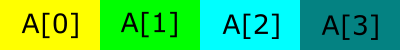The original Array A consists of four elements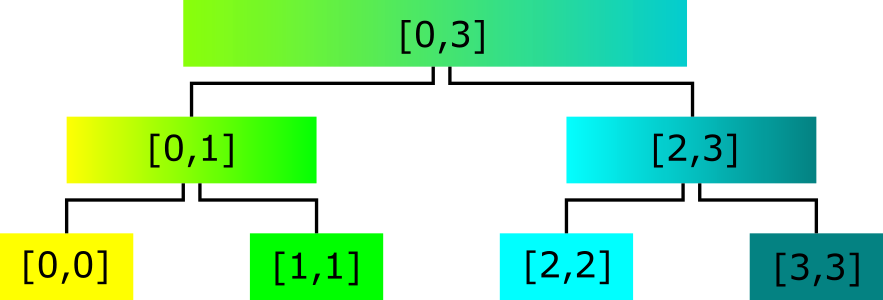Segment tree that stores the results of various intervals of the array A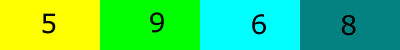Pictorial representation of array A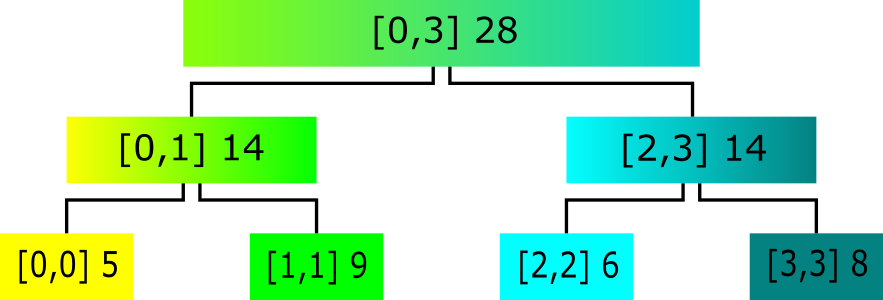The range sum segment tree for array A with intervals and results.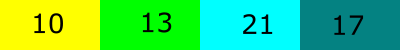Pictorial representation of array A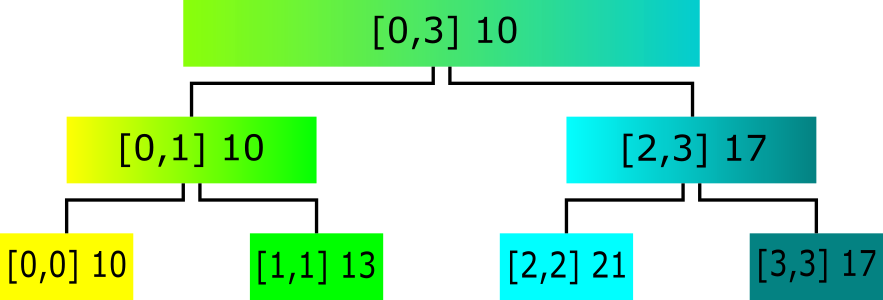The range min segment tree for array A with intervals and results.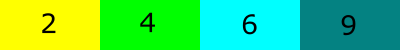Pictorial representation of array A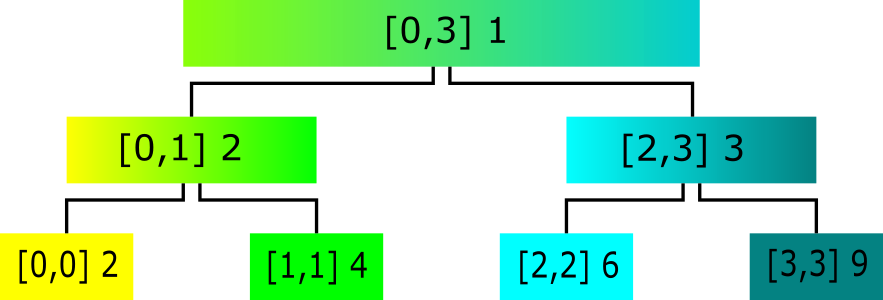The range gcd segment tree for array A with intervals and results.

# Segment tree : Array representation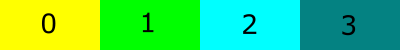An array with 4 elements has the indexes 0,1,2,3.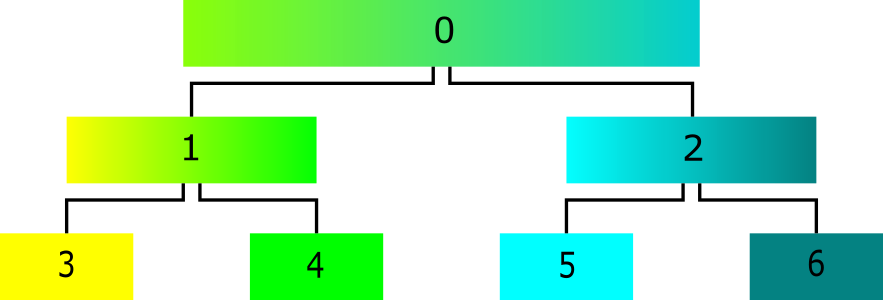The corresponding segment tree for the array in the image above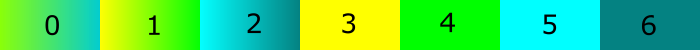Representing the segment array in form of an array